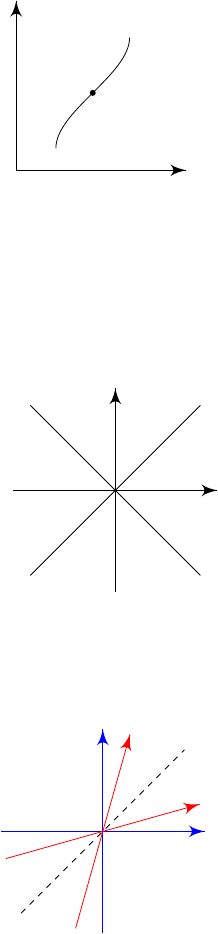8Special relativity

IA Dynamics and Relativity8.2 Spacetime diagrams
It is often helpful to plot out what is happening on a diagram. We plot them on
a graph, where the position
x
is on the horizontal axis and the time
ct
is on the
vertical axis. We use ct instead of t so that the dimensions make sense.
x
ct
world line
P
Definition
(Spacetime)
.
The union of space and time in special relativity is
called Minkowski spacetime. Each point
P
represents an event, labelled by
coordinates (ct, x) (note the order!).
A particle traces out a world line in spacetime, which is straight if the particle
moves uniformly.
Light rays moving in the x direction have world lines inclined at 45
.
x
ct
light raylight ray
We can also draw the axes of
S
0
, moving in the
x
direction at velocity
v
relative to
S
. The
ct
0
axis corresponds to
x
0
= 0, i.e.
x
=
vt
. The
x
0
axis
corresponds to t
0
= 0, i.e. t = vx/c
2
.
x
ct
x
0
ct
0
Note that the
x
0
and
ct
0
axes are not orthogonal, but are symmetrical about
the diagonal (dashed line). So they agree on where the world line of a light ray
should lie on.